## You are standing on a slippery sheet of ice that covers a parking lot. A friend throws you a 0.600‐kg ball that is traveling horizontally at

Question

You are standing on a slippery sheet of ice that covers a parking lot. A friend throws you a 0.600‐kg ball that is traveling horizontally at 10.0 m/s. Your mass is 70.0 kg. a) If you catch the ball, with what speed will you and the ball move afterward? b) If the ball hits you and bounces off of your chest, so afterward it is moving horizontally at 8.0 m/s in the opposite direction, what is your speed after the collision?

in progress 0
6 months 2021-07-27T14:30:10+00:00 1 Answers 6 views 0

(a). The final speed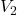= 0.085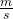(b). The speed after collision= 0.15Explanation: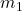= 0.6 kg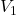= 10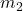= 70 + 0.6 = 70.6  kg

(a). From conservation of momentum principal=0.6 × 10 = 70.6 ×= 0.085This is the final speed.

(b). From conservation of momentum principal

0.6 × 10 = 0.6 × (-8) + 7070= 6 + 4.8= 0.15This is the speed after collision.